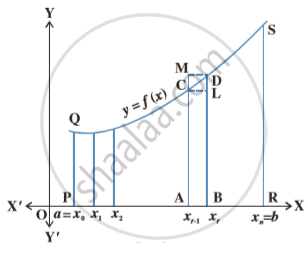# Definite Integral as the Limit of a Sum

#### notes

Let f be a continuous function defined on close interval [a, b]. Assume that all the values taken by the function are non negative, so the graph of the function is a curve above the x-axis.
The definite integral int_a^bf(x) dx  is the area bounded by the curve y = f(x), the ordinates x = a, x = b and the x-axis. To evaluate this area, consider the region PRSQP between this curve, x-axis and the ordinates x = a and x = b. Fig.Divide the interval [a, b] into n equal subintervals denoted by [x_0, x_1], [x_1, x_2] ,..., [x_(r – 1), x_r], ..., [x_(n – 1), x_n], where  x_0 = a, x_1 = a + h, x_2 = a + 2h, ... , x_r = a + rh and x_n = b = a + nh or n =(b-a)/h We note that as n → ∞, h → 0.  From the above fig. we have
area of the rectangle (ABLC) < area of the region (ABDCA) < area of the rectangle (ABDM)        ... (1)
Same  as x_r – x_(r–1) → 0, i.e., h → 0 all the three areas shown in (1) become nearly equal to each other. Now we form the following sums.

s_n = h[f(x_0) + ...+f(x_(n-1))] = h summation f(x_r)   ...(2)
and S_n =h[f(x_1) + f(x_2) + ...+ f(x^n)] = h summation f(x_r)    ..(3)
Here, s_n and S_n denote the sum of areas of all lower rectangles and upper rectangles raised over subintervals [x_(r–1), x_r] for r = 1, 2, 3, …, n, respectively. In view of the inequality (1) for an arbitrary subinterval [x_(r–1), x_r], we have s_n < area of the region PRSQP < S_n                ... (4)
As n→∞ strips become narrower and narrower, it is assumed that the limiting values of (2) and (3) are the same in both cases and the common limiting value is the required area under the curve.
Symbolically, we write
lim_(n ->∞ ) S_n = lim _(n-> ∞) s_n =  area of the region PRSQP = int _a^b f(x)
dx         ...(5)
It follows that this area is also the limiting value of any area which is between that of the rectangles below the curve and that of the rectangles above the curve.
int _a^b f(x)dx =lim_(h->0) h [f(a) + f(a+h) + ...+f(a+(n-1))h]
or int _a^b f(x) dx = (b-a) lim_(n ->∞) 1/ n [f(a) + f(a+h) + ...+ f(a+(n-1)h)] ...(6)
where h = (b-a)/n -> 0 as n -> ∞

Remark: The value of the definite integral of a function over any particular interval depends on the function and the interval, but not on the variable of integration that we choose to represent the independent variable. If the independent variable is denoted by t or u instead of x, we simply write the integral as int _a^b f(t)dt or int _a^b f(u)du instead of  int _a^b f(x)dx. Hence, the variable of integration is called a dummy variable.

If you would like to contribute notes or other learning material, please submit them using the button below.

#### Video Tutorials

We have provided more than 1 series of video tutorials for some topics to help you get a better understanding of the topic.

Series 1

Series 2

Series 3

### Shaalaa.com

Definite Integral as Limits of Sum [00:13:19]
S
0%